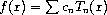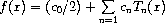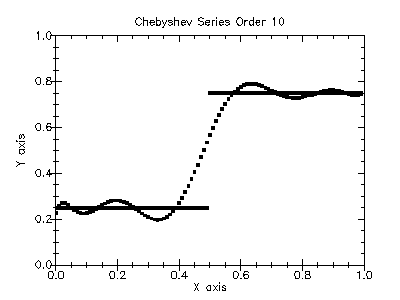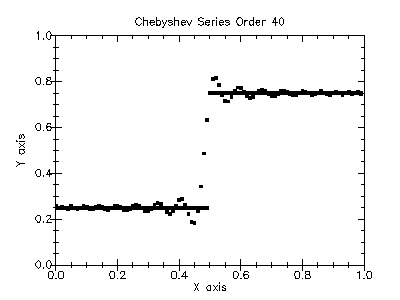Version: 4.2.1

### 11Chebyshev Approximations

This chapter describes the routines for computing Chebyshev approximations to univariate functions provided by the PLT Scheme Science Collection. A Chebyshev approximation is a truncation of the serieswhere the Chebyshev polynomials Tn(x) = cos(n arccos x) provides an orthogonal basis of polynomials in the interval [-1, 1] with the weight function 1/(1 - x2)½. The first few Chebyshev polynomials are T0(x) = 1, T1(x) = x, T2(x) = 2x2 -1. For more information, see Abramowitz and Stegun [Abramowitz64], Chapter 22.

The functions described in this chapter are defined in the "chebyshev.ss" file in the science collection are are made available using the form:

 (require (planet williams/science/chebyshev))

#### 11.1The chebyshev-series Structure

A Chebyshev series is represented by a structure chebyshev-series that has the following fields:

• coefficients – a vector of length order containing the coefficients for the Chebyshev series.

• order – the order of the Chebyshev series.

• lower – the lower bound on the interval over which the Chebyshev series is defined.

• upper – the upper bound on the interval over which the Chebyshev series is defined.

The approximations are made over the range [lower, upper] using order + 1 terms, including coefficient0. The series is computed using the following convention:which is needed when accessing the coefficients directly.

#### 11.2Creation and Calculation of Chebyshev Series

 (make-chebyshev-series order) → chebyshev-series? order : exact-nonnegative-integer?
Returns a newly created Chebyshev series with the given order.

 (chebyshev-series-coefficients cs) → (vectorof real?) cs : chebyshev-series?
Returns the coefficients field of the Chebyshev series cs.

 (chebyshev-series-order cs) → exact-nonnegative-integer? cs : chebyshev-series?
Returns the order field of the Chebyshev series cs.

 (chebyshev-series-lower cs) → real? cs : chebyshev-series?
Returns the lower field of the Chebyshev series cs.

 (chebyshev-series-upper cs) → real? cs : chebyshev-series?
Returns the upper field of the Chebyshev series cs.

 (chebyshev-series-init cs func a b) → void? cs : chebyshev-series? func : (-> real? real?) a : real? b : (>/c a)
Computes the Chebyshev approximation cs for the function func over the range (a, b) to the previously specified order. The computation of the Chebyshev approximation is an O(n2) process that requires n function evaluations.

#### 11.3Chebyshev Series Evaluations

 (chebyshev-eval cs x) → real? cs : chebyshev-series? x : real?
Evaluates the Chebyshev series cs at the given point x.

 (chebyshev-eval-n cs n x) → real? cs : chebyshev-series? n : exact-nonnegative-integer? x : real?
Evaluates the Chebyshev series cs at the given point x to (at most) the given order n.

#### 11.4Derivatives and Integrals

The following functions allow a Chebyshev series to be differentiated or integrated, producing a new Chebyshev series.

 (make-chebyshev-series-derivative series) → chebyshev-series? series : chebyshev-series?
Returns a new Chebyshev series object that is the derivative of series. The returned series has the same order and range as series.

 (make-chebyshev-series-integral series) → chebyshev-series? series : chebyshev-series?
Returns a new Chebyshev series object that is the integral of series. The returned series has the same order and range as series.

#### 11.5Chebyshev Approximation Examples

Example: The following program computes Chebyshev approximations to a step function. This is an extremely difficult approximation to make due to the discontinuity and was chosen as an example where approximation error is visible. For smooth functions the Chebyshev approximation converges extremely rapidly and errors would not be visible.

 #lang scheme (require (planet williams/science/chebyshev)) (require plot/plot) (define (f x) (if (< x 0.5) 0.25 0.75)) (define (chebyshev-example n) (let ((cs (make-chebyshev-series-order 40)) (y-values '()) (y-cs-10-values '()) (y-cs-40-values '())) (chebyshev-series-init cs f 0.0 1.0) (do ((i 0 (+ i 1))) ((= i n) (void)) (let* ((x (exact->inexact (/ i n))) (y (f x)) (y-cs-10 (chebyshev-eval-n cs 10 x)) (y-cs-40 (chebyshev-eval cs x))) (set! y-values (cons (vector x y) y-values)) (set! y-cs-10-values (cons (vector x y-cs-10) y-cs-10-values)) (set! y-cs-40-values (cons (vector x y-cs-40) y-cs-40-values)))) (printf "~a~n" (plot (mix (points (reverse y-values)) (points (reverse y-cs-10-values))) (x-min 0) (x-max 1) (y-min 0) (y-max 1) (title "Chebyshev Series Order 10"))) (printf "~a~n" (plot (mix (points (reverse y-values)) (points (reverse y-cs-40-values))) (x-min 0) (x-max 1) (y-min 0) (y-max 1) (title "Chebyshev Series Order 40"))))) (chebyshev-example 100)

The following figures show the resulting plots.